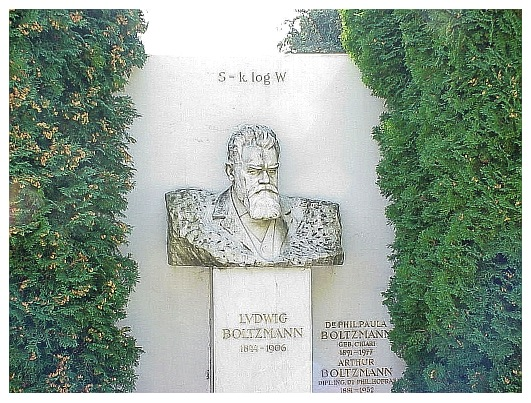## Friday, September 18, 2015

### Boltzmann’s TombAsimov’s Biographical Encyclopedia of Science and Technology, by Isaac Asimov.
In Chapter 3 of the 5th edition of Intermediate Physics for Medicine and Biology, Russ Hobbie and I discuss the Boltzmann factor and Boltzmann’s constant. Later in the book, we analyze the Poisson-Boltzmann equation and the Stefan-Boltzmann law. Who was Boltzmann? In Asimov’s Biographical Encyclopedia of Science and Technology (2nd revised edition), Isaac Asimov writes
BOLTZMANN, Ludwig Edward (bohlts’mahn)
Austrian physicist
Born: Vienna, February 20, 1844
Died: Duino, near Trieste (then in Austria, now in Italy), September 5, 1906

Boltzmann, the son of a civil servant, received his Ph.D. from the University of Vienna in 1866. His work on the kinetic theory of gases was done independently of Maxwell and they share the credit.

Beginning in 1871, Boltzmann increased the rigor of the mathematical treatment and emphasized the statistical interpretation of the second law of thermodynamics thus founding “statistical mechanics.” He showed that Clausius’ concept of increasing entropy of disorder [could be based on statistical ideas], laying the groundwork for the later achievements of Gibbs.

He was a firm proponent of atomism at a time when Ostwald was mounting the final campaign against it. Boltzmann also advanced a mathematical treatment that explained the manner in which, according to the experimental observations of Stefan (whom Boltzmann, in this college years, served as assistant), quantity of radiation increased as the fourth power of the temperature. This is therefore sometimes called the Stefan-Boltzmann law.

Boltzmann turned down a chance to succeed Kirchhoff at Berlin but in 1894 succeeded to Stefan’s post in Vienna.

Though Boltzmann lived longer than Maxwell, his life too was cut short. In his case it was suicide, brought on by recurrent episodes of severe mental depression accentuated, perhaps, by opposition to his atomistic notions by Oswald and others.

His equation relating entropy and disorder was engraved on the headstone of his grave.
I particularly am intrigued by the last sentence of Asimov’s entry. Who puts an equation on their tombstone? Boltzmann did!Boltzmann's tombstone.
This equation is Eq. 3.20 in IPMB.

S = kB ln Ω ,

where S is the entropy, kB is Boltzmann’s constant, ln is the natural logarithm, and Ω is the number of microstates. The equation says that the entropy increases as the number of possible microstates increases. If there are only one or a few states available, the entropy is small; if there are many states available, the entropy is large. Thus, from a statistical mechanics point-of-view, the thermodynamic concept of entropy (developed well before Boltzmann’s work) is a measure of the number of states. The logarithm is important, because if system A has 10 states available and system B has 20 states available, the total number of states is the product, 200. If the entropy were proportional to Ω, the total entropy of the two systems would not be the sum of the entropy in each system. However, the logarithm property ln(ΩAΩB)=ln(ΩA)+ln(ΩB) ensures that the entropy is indeed additive.

The definition of entropy in terms of the number of states is a fundamental relationship connecting thermodynamics and statistical mechanics. No wonder Boltzmann wanted it on his tombstone.